# The STATESPACE Procedure

### Parameter Estimation

The model is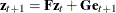, where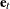is a sequence of independent multivariate normal innovations with mean vector 0 and variance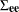. The observed sequence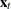composes the first r components of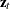, and thus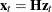, where H is thematrix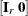.

Let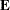be thematrix of innovations: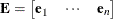If the number of observations n is reasonably large, the log likelihood L can be approximated up to an additive constant as follows: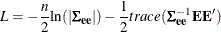The elements of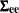are taken as free parameters and are estimated as follows: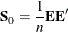Replacingby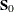in the likelihood equation, the log likelihood, up to an additive constant, is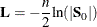Letting B be the backshift operator, the formal relation betweenandis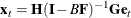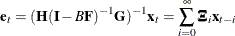Letting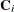be the ith lagged sample covariance ofand neglecting end effects, the matrixis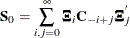For the computation of, the infinite sum is truncated at the value of the KLAG= option. The value of the KLAG= option should be large enough that the sequence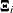is approximately 0 beyond that point.

Let
be the vector of free parameters in the
andmatrices. The derivative of the log likelihood with respect to the parameteris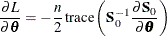The second derivative is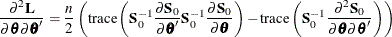Near the maximum, the first term is unimportant and the second term can be approximated to give the following second derivative approximation: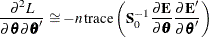The first derivative matrix and this second derivative matrix approximation are computed from the sample covariance matrix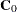and the truncated sequence. The approximate likelihood function is maximized by a modified Newton-Raphson algorithm that employs these derivative matrices.

The matrixis used as the estimate of the innovation covariance matrix,. The negative of the inverse of the second derivative matrix at the maximum is used as an approximate covariance matrix for the parameter estimates. The standard errors of the parameter estimates printed in the parameter estimates tables are taken from the diagonal of this covariance matrix. The parameter covariance matrix is printed when the COVB option is specified.

If the data are nearly nonstationary, a better estimate ofand the other parameters can sometimes be obtained by specifying the RESIDEST option. The RESIDEST option estimates the parameters by using conditional least squares instead of maximum likelihood.

The residuals are computed using the state space equation and the sample mean values of the variables in the model as start-up values. The estimate ofis then computed using the residuals from the ith observation on, where i is the maximum number of times any variable occurs in the state vector. A multivariate Gauss-Marquardt algorithm is used to minimize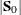. See Harvey (1981a) for a further description of this method.